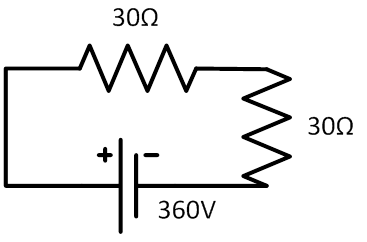### Sample ProblemWhat is the total power of the two resistors in this circuit?

#### Solution

P = IV = V2/R

P =  (360V) 2 ÷ (30Ω + 30 Ω)  = 2160 Watt